Test: Sampling Theorem and Nyquist Rate

# Test: Sampling Theorem and Nyquist Rate - Electrical Engineering (EE)

Test Description

## 10 Questions MCQ Test GATE Electrical Engineering (EE) 2024 Mock Test Series - Test: Sampling Theorem and Nyquist Rate

Test: Sampling Theorem and Nyquist Rate for Electrical Engineering (EE) 2023 is part of GATE Electrical Engineering (EE) 2024 Mock Test Series preparation. The Test: Sampling Theorem and Nyquist Rate questions and answers have been prepared according to the Electrical Engineering (EE) exam syllabus.The Test: Sampling Theorem and Nyquist Rate MCQs are made for Electrical Engineering (EE) 2023 Exam. Find important definitions, questions, notes, meanings, examples, exercises, MCQs and online tests for Test: Sampling Theorem and Nyquist Rate below.
Solutions of Test: Sampling Theorem and Nyquist Rate questions in English are available as part of our GATE Electrical Engineering (EE) 2024 Mock Test Series for Electrical Engineering (EE) & Test: Sampling Theorem and Nyquist Rate solutions in Hindi for GATE Electrical Engineering (EE) 2024 Mock Test Series course. Download more important topics, notes, lectures and mock test series for Electrical Engineering (EE) Exam by signing up for free. Attempt Test: Sampling Theorem and Nyquist Rate | 10 questions in 10 minutes | Mock test for Electrical Engineering (EE) preparation | Free important questions MCQ to study GATE Electrical Engineering (EE) 2024 Mock Test Series for Electrical Engineering (EE) Exam | Download free PDF with solutions
 1 Crore+ students have signed up on EduRev. Have you?
Test: Sampling Theorem and Nyquist Rate - Question 1

### The highest frequency component of a speech signal needed for telephonic communications is about 3.1 kHz. What is the suitable value for the sampling rate?

Detailed Solution for Test: Sampling Theorem and Nyquist Rate - Question 1

Nyquist Sampling Theorem:
A continuous-time signal can be represented in its samples and can be recovered back when sampling frequency fs is greater than or equal to twice the highest frequency component of the message signal, i.e.
fs ≥ 2fm
Therefore when we want to convert continuous signals to discrete signals, the sampling must be done at the Nyquist rate.
Calculation:
Given that,
fm = 3.1 kHz
⇒ fs ≥ 2fm
⇒ fs ≥ 2 × 3.1 = 6.4 kHz

Test: Sampling Theorem and Nyquist Rate - Question 2

### A single tone 4 kHz message signal is sampled with 9 kHz, 7 kHz and 5 kHz.  Aliasing effect will be seen in the reconstructed signal, when the signal is sampled with

Detailed Solution for Test: Sampling Theorem and Nyquist Rate - Question 2

Concept:
The sampling theorem states that “a signal can be exactly reproduced if it is sampled at the rate fs which is greater than twice the maximum frequency W in the modulating signal."
If the sampled frequency is less than the Nyquist frequency, overlapping of lower and upper sidebands known as aliasing takes place.
The main reason for aliasing is under-sampling, i.e. sampling the signal at a frequency less than twice the Nyquist rate.
fs < 2fm
fs = Sampling frequency
fm = Modulating frequency
Application:
The minimum sampling rate to avoid aliasing will be:
fs = 2 × 2fm = 2 × 4 kHz
fs = 8 kHz
∴ For fs ≥ 8 kHz, the sampling frequency will satisfy the Nyquist rate and no aliasing will take place.
And for both 7 kHz and 5 kHz sampling rates, the Aliasing effect will be seen in the reconstructed signal as the sampling frequency is less than the Nyquist Rate.

Aliasing is explained with the help of the spectrum as shown: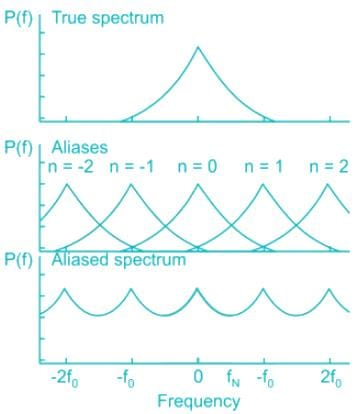Test: Sampling Theorem and Nyquist Rate - Question 3

### The figure given below shows the Fourier spectra of signal x(t) and y(t).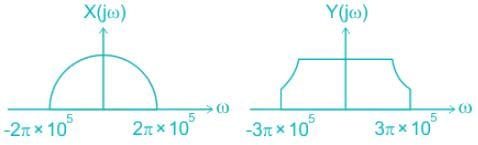The Nyquist sampling rate for x(t) y(t) is_________.

Detailed Solution for Test: Sampling Theorem and Nyquist Rate - Question 3

Concept:
Multiplication in the time domain is the convolution in the frequency domain and the maximum frequency of convolution of the signal is the sum of individual frequencies, i.e.
If z(t) = x(t) y(t)
The maximum frequency of Z(ω) = ωx + ωy
where ωx is the maximum frequency of signal x(t)
ωy is the maximum frequency of signal y(t)
Application:
The maximum frequency present in x(t) is: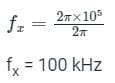Similarly, the maximum frequency present in y(t) is: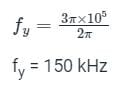Now, the maximum frequency present in z(t) = x(t) y(t) will be:
fz = fx + fy
fz = 250 kHz
Nyquist sampling frequency will now be:
fN = 2 × fz
fN = 500 kHz

Test: Sampling Theorem and Nyquist Rate - Question 4

Consider the signal x(t) = cos(6πt) + sin(8πt), where t is in seconds. The Nyquist sampling rate (in samples/second) for the signal y(t) = x(2t + 5) is

Detailed Solution for Test: Sampling Theorem and Nyquist Rate - Question 4

Given,
x(t) = cos(6πt) + sin(8πt)
The bandwidth of the signal will be: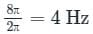Now, y(t) = x(2t + 5) can be written as: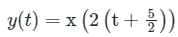Taking the Fourier transform, we get: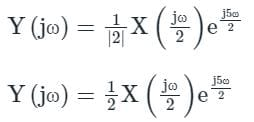Thus, the frequency spectrum of X(jω)  expands by 2.
This will make the highest frequency component of Y(jω) as:
2 × 4 = 8 Hz
Hence, the Nyquist rate will be:
fs = 2 × 8 = 16 samples/sec

Test: Sampling Theorem and Nyquist Rate - Question 5

A continuous-time signal has frequency content at f = 10 MHz, 50 MHz, and 70 MHz. The signal is sampled at a sampling frequency of 56 MHz and is then passed through a low-pass filter with a cutoff frequency of 15 MHz. The frequency content of the output of the filter will be:

Detailed Solution for Test: Sampling Theorem and Nyquist Rate - Question 5

Calculation:
fs = Sampling Frequency = 56 MHz
The frequencies present at the input are:
fm1 = 10 MHz, fm2 = 50 MHz and, fm3 = 70 MHz
Once sampled, the frequency content at the output will be: fm ± fs
For, fm1 = 10 MHz, the output will contain frequencies of,
= fm1, fm1 ±, fm1 ± 2fs ….
= 10 MHz, 66 MHz, 122 MHz
For, fm2 = 50 MHz, the output will contain frequencies of
= fm2, fm2 ± fs, fm2 ± 2fs ….
= 50 MHz, 6 MHz, 106 MHz, 162 MHz
For, fm3 = 70 MHz, the output will contain frequencies of
= fm3, fm3 ± fs, fm3 ± 2fs ….
= 70 MHz, 14 MHz, 182 MHz ….
When these frequencies are passed through a low pass filter with a cutoff frequency of 15 MHz, the only remaining frequencies at its output will be 6MHz, 10 MHz and 14 MHz.

Test: Sampling Theorem and Nyquist Rate - Question 6

A sinusoid x(t) of unknown frequency is sampled by an impulse train of period 20 ms. The resulting sample train is next applied to an ideal lowpass filter with cutoff at 25 Hz. The filter output is seen to be a sinusoid of frequency 20 Hz. This means that x(t) is

Detailed Solution for Test: Sampling Theorem and Nyquist Rate - Question 6

Period of sampling train, Ts = 20 ms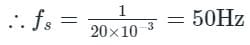If frequency of x(t) is fx, then after sampling the signal, the sampled signal has the frequency,
fs - fx = 50 - fx and fs + fx = 50 + fs
Now, the sampled signal is applied to and ideal low pass filter with cut off frequency.
fc = 25 Hz
Now, the o/p of filter carried a single frequency component of 20 Hz
∴, only (fs−fx) component passes through the filter, ie.
fs - fx < 25
and fs - fx = 20
50 - fx­ = 20
fx = 50 - 20 = 30 Hz

Test: Sampling Theorem and Nyquist Rate - Question 7

An input signal x(t) = 2 + 5sin⁡(100πt) is sampled with a sampling frequency of 400 Hz and applied to the system whose transfer function is represented by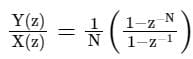where, N represents the number of samples per cycle. The output y[n] of the system under steady state is

Detailed Solution for Test: Sampling Theorem and Nyquist Rate - Question 7

Concept:
Final value theorem,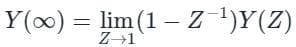Calculation:
We have: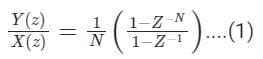and x(t) = 2 + 5sin(100πt)
sampling frequency, fs = 400 Hz
put t = nTs, the output of the sampling process is,
x(nTs) = 2 + sin(100πnTs)
x(nTs) = 2 + 5 sin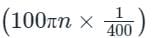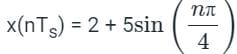where,
ω0 = π/4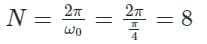The z-transform of x(n) is,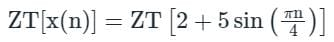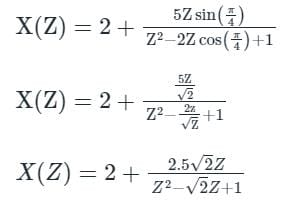From equation (i)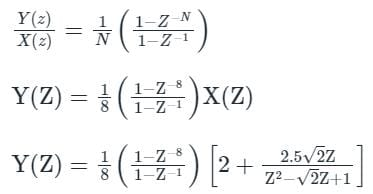using final value theorem,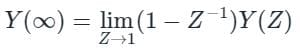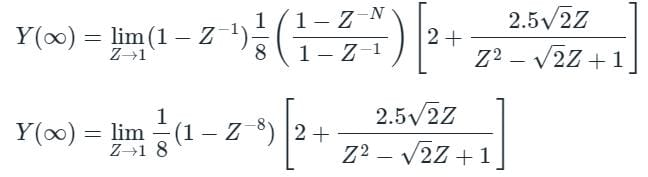y(∞) = 0

Test: Sampling Theorem and Nyquist Rate - Question 8

If a 100 Hz sinusoidal signal is sampled at the rates of 140 Hz, 90 Hz, and 30 Hz, then the aliased frequencies correspond to each sampling rate will be respectively:

Detailed Solution for Test: Sampling Theorem and Nyquist Rate - Question 8

Concept:
Aliased frequency component is given by:
fa = |fs – fm|
fs = sampling frequency
fm = message signal frequency

Calculation:
For a sampling rate of 140 Hz, since fs > fm:
Aliased Frequency = fs - fm,
= 140 – 100 = 40 Hz
For a sampling rate of 90 Hz, since fs < fm:
Aliased Frequency = fm – fs,
= 100 – 90 = 10 Hz
For a sampling rate of 30 Hz, since fs < fm:
Aliased Frequency = fm – fs
= 100 – 30 = 70 Hz

Aliasing is explained with the help of the spectrum as shown: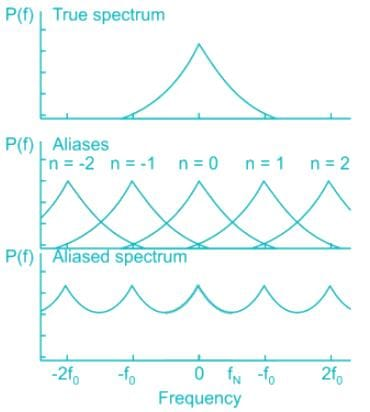Test: Sampling Theorem and Nyquist Rate - Question 9

The Nyquist sampling interval, for the signal sinc(700t) + sinc(500t) is:

Detailed Solution for Test: Sampling Theorem and Nyquist Rate - Question 9

Concept:
Nyquist rate: The minimum sampling rate is often called the Nyquist rate. The Nyquist sampling rate is two times the highest frequency of the input or message signal.
fs = 2fm
Where,
fs is the minimum sampling frequency or Nyquist rate
fm is the highest frequency of the input or message signal.
Calculation:
sinc(700t) + sinc(500t)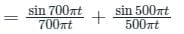f1 = 700π/2π = 350 Hz
f2 = 500π/2π = 250 Hz
fm = max (f1, f2) = max (350, 250) = 350 Hz
Sampling frequency,
fs = 2fm = 2 × 350 = 700 Hz
Sampling time period = 1/700 sec

Test: Sampling Theorem and Nyquist Rate - Question 10

A band-limited signal with a maximum frequency of 5 kHz is to be sampled. According to the sampling theorem, the sampling frequency which is not valid is

Detailed Solution for Test: Sampling Theorem and Nyquist Rate - Question 10

The maximum frequency of the band-limited signal.
fm = 5 kHz
According to the Nyquist sampling theorem, the sampling frequency must be greater than the Nyquist  frequency which is given as
fN = 2 fm = 2 × 5 = 10 kHz
So the sampling frequency fs ≥ fN
fs ≥ 10 kHz
Only the option (A) does not satisfy the condition.
∴ 5 kHz is not a valid sampling frequency.

## GATE Electrical Engineering (EE) 2024 Mock Test Series

23 docs|285 tests
Information about Test: Sampling Theorem and Nyquist Rate Page
In this test you can find the Exam questions for Test: Sampling Theorem and Nyquist Rate solved & explained in the simplest way possible. Besides giving Questions and answers for Test: Sampling Theorem and Nyquist Rate, EduRev gives you an ample number of Online tests for practice

## GATE Electrical Engineering (EE) 2024 Mock Test Series

23 docs|285 tests